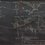# Salvaging proofs

I botched up a proof, but I salvaged useful bits and bobs from it.

We prove $\zeta^2(s) < \zeta(s+1)\zeta(s-1)$ by recalling the Dirichlet series of $\phi$, the Euler totient function, that is,

$\sum_{n=1}^{\infty} \frac{\phi(n)}{n^s} = \frac{\zeta(s-1)}{\zeta(s)}$

Now we know that, for all $n \geq 2$,

$\frac{\phi(n)}{n^{s+1}} < \frac{\phi(n)}{n^s} \longrightarrow \sum_{n=2}^{\infty} \frac{\phi(n)}{n^{s+1}} < \sum_{n=2}^{\infty} \frac{\phi(n)}{n^s} \quad \therefore \frac{\zeta(s)}{\zeta(s-1)} < \frac{\zeta(s+1)}{\zeta(s)}$

$\therefore \zeta^2(s) < \zeta(s+1)\zeta(s-1)$

Personally, I think it is interesting, as it invokes a Dirichlet series in a somewhat unexpected way. Also, this implies that $\lbrace \frac{\zeta(n+1)}{\zeta(n)} \rbrace _{n=2}$ is monotonically increasing.

Challenge: Prove $2\zeta(s) < \zeta(s+1)+\zeta(s-1)$, using the above result or by the trivial inequality.Note by Jake Lai
5 years, 9 months ago

This discussion board is a place to discuss our Daily Challenges and the math and science related to those challenges. Explanations are more than just a solution — they should explain the steps and thinking strategies that you used to obtain the solution. Comments should further the discussion of math and science.

When posting on Brilliant:

• Use the emojis to react to an explanation, whether you're congratulating a job well done , or just really confused .
• Ask specific questions about the challenge or the steps in somebody's explanation. Well-posed questions can add a lot to the discussion, but posting "I don't understand!" doesn't help anyone.
• Try to contribute something new to the discussion, whether it is an extension, generalization or other idea related to the challenge.

MarkdownAppears as
*italics* or _italics_ italics
**bold** or __bold__ bold
- bulleted- list
• bulleted
• list
1. numbered2. list
1. numbered
2. list
Note: you must add a full line of space before and after lists for them to show up correctly
paragraph 1paragraph 2

paragraph 1

paragraph 2

[example link](https://brilliant.org)example link
> This is a quote
This is a quote
    # I indented these lines
# 4 spaces, and now they show
# up as a code block.

print "hello world"
# I indented these lines
# 4 spaces, and now they show
# up as a code block.

print "hello world"
MathAppears as
Remember to wrap math in $$ ... $$ or $ ... $ to ensure proper formatting.
2 \times 3 $2 \times 3$
2^{34} $2^{34}$
a_{i-1} $a_{i-1}$
\frac{2}{3} $\frac{2}{3}$
\sqrt{2} $\sqrt{2}$
\sum_{i=1}^3 $\sum_{i=1}^3$
\sin \theta $\sin \theta$
\boxed{123} $\boxed{123}$

Sort by:

Since if $a,b>0$, $a^{2}+b^{2}>2ab$

$2\zeta (s+1)\zeta (s-1)<{ \zeta (s+1) }^{ 2 }+{ \zeta (s-1) }^{ 2 }$

Following the note's inequality, $2{ \zeta (s) }^{ 2 }<2\zeta (s+1)\zeta (s-1)<{ \zeta (s+1) }^{ 2 }+{ \zeta (s-1) }^{ 2 }$ $4{ \zeta (s) }^{ 2 }<{ \zeta (s+1) }^{ 2 }+{ \zeta (s-1) }^{ 2 }+2\zeta (s+1)\zeta (s-1)$ $2\zeta (s)<{ \zeta (s+1) }+{ \zeta (s-1) }$

- 5 years, 9 months ago

Or that. I think that spotting that there's a way to slip in AM-GM is easier, but again, that just depends on what prior experience one has.

There is also a proof without the result of the challenge by the trivial inequality, which is kind of obvious.

- 5 years, 9 months ago

I think you meant $\lbrace \frac{\zeta(n+1)}{\zeta(n)} \rbrace _{n\ge2}$

- 5 years, 9 months ago

Same thing, hehe.

- 5 years, 9 months ago

Maybe I misunderstood the notation...

- 5 years, 9 months ago

Yours and mine both mean the same thing. I've seen both used before, and they all mean what we're both trying to say.

- 5 years, 9 months ago

Just prove that $\zeta(s)$ concave downwards, the inequality follows.

- 5 years, 9 months ago

I just realised, Cauchy-Schwarz on $\ell^2$ works.

- 5 years, 7 months ago